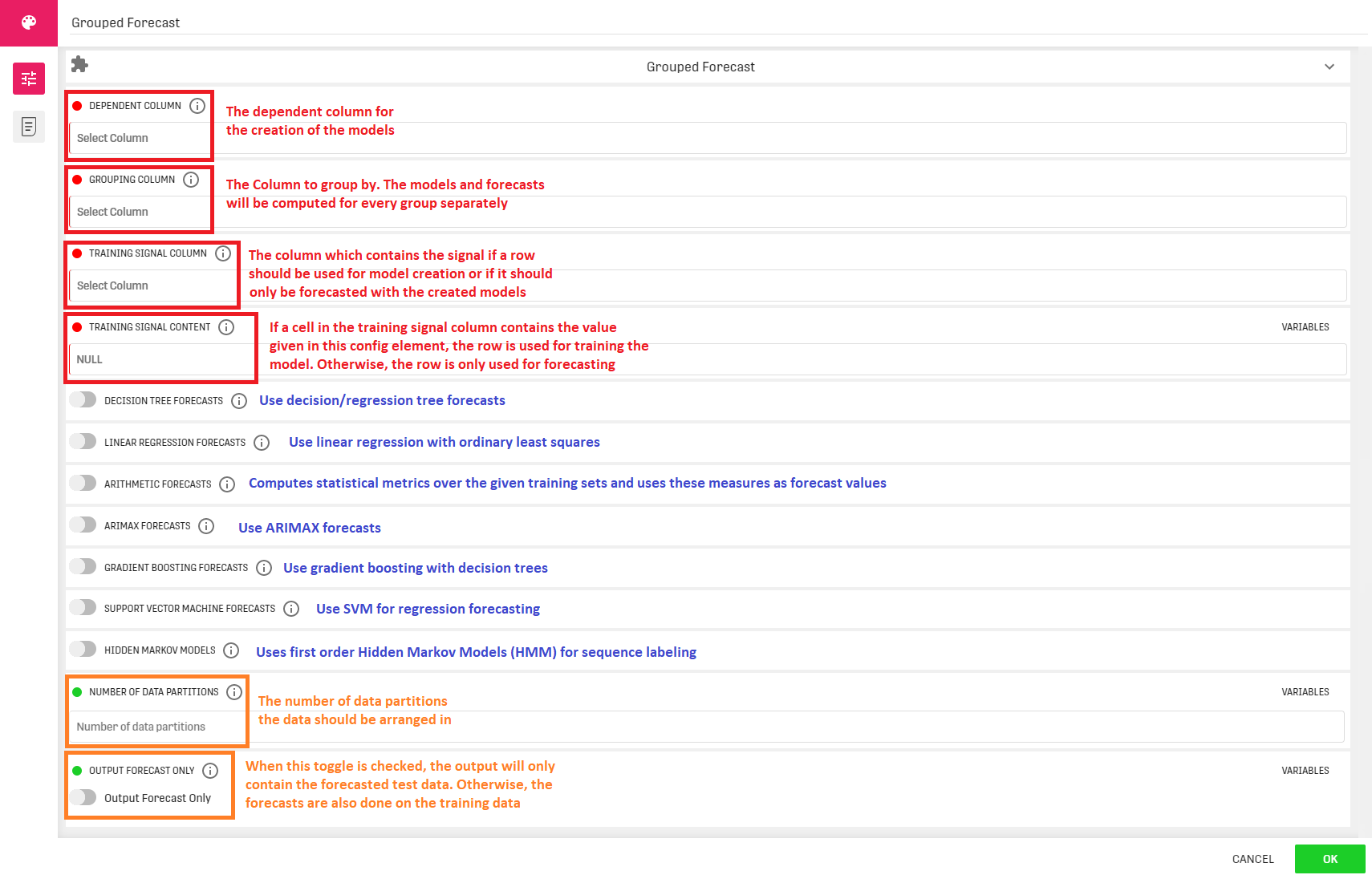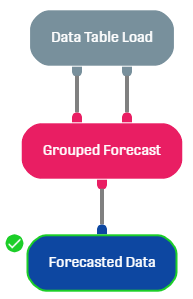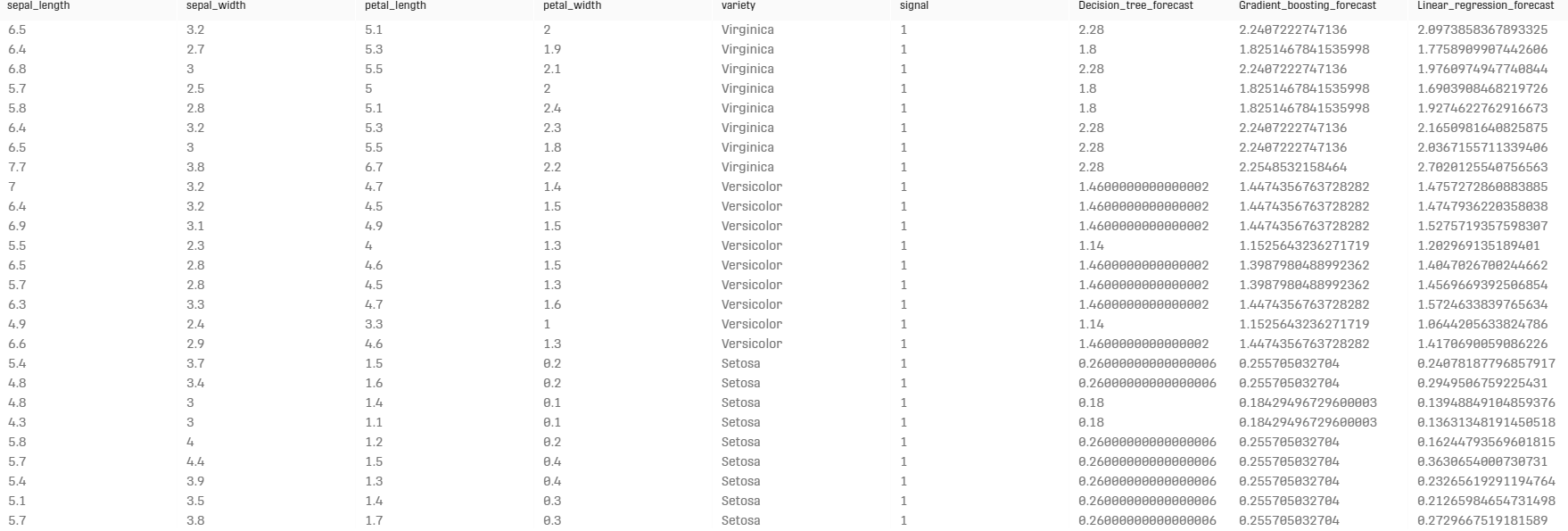# Overview

The Grouped Forecast Processor uses multiple decision methods for creating arbitrary forecasts on grouped data series.

# Input

The processor has two input ports. The first input contains relevant data, training and test datasets are combine into one dataset and each type is referred to by a signal column. The second input is currently not used, it can be connected to some arbitrary data (e.g. empty table).

Note that the relevant input data should contain one grouping column. The models and forecasts will be computed for every group separately.

# ConfigurationThe available forecasts methods are the following (links for further information are also provided):

• Decision tree: Decision/Regression trees are automatically selected depending on the type of the dependent column: text, timestamp and integer columns lead to a decision tree, double and numeric columns lead to a regression tree.
• Linear regression: Predict a target variable by fitting the best linear relationship between the dependent and independent variable.
• Arithmetic forecasts: Computes statistical metrics over the given training sets and uses these measures as forecast values, e.g. by using "Average" as forecast function, all to-be-forecasted data will have the average of the training dataset as forecasted value.
• ARIMAX forecasts: Fits an AR(I)MA (Autoregressive (Integrated) Moving Average) or AR(I)MAX (AR(I)MA with exogenous regressors) model, where the latter includes exogenous variables that must be provided by the user as additional attributes. An AR(I)MA model is determined by an intercept, the number of autoregressive coefficients (p), the number of moving average coefficients (q) and the differencing order d. Moreover, the user can select if the model includes an intercept or a trend. For ARIMAX models with differencing order d > 0 and exogenous regressors, the intercept is interpreted as a stochastic trend, also called drift.
• Gradient boosting: Boosting is an ensemble technique in which the predictors are not made independently, but sequentially.
• SVM forecasts: A discriminative classifier formally defined by a separating hyperplane.
• Hidden Markov Models: Uses first order Hidden Markov Models (HMM) for sequence labeling. Each group determined by the grouping column is assumed to have multiple sequences of which some are marked with the training signal. The transition, emission and initial probabilities are estimated using maximum likelihood estimation.

# Output

The input dataset is forwarded to the output port, an additional column for every used forecast method is added along with the forecasted values for the dependent variable. When the "Output forecast only" toggle is checked, the output will only contain the forecasted test data (specified by the signal column). Otherwise, the forecasts are also done on the training data.

# Example

In this example, the iris dataset is used along with an additional column called "signal" specifying whether the corresponding line is used for training or testing. The forecasted variable is the "petal_width" and the result is grouped by the variable "variety".

## Workflowgiven that the second input for the grouped forecast processor is irrelevant, we use the invalid rows result from the data table load processor as input.

## ResultThe previous result could be associated to the Forecast Method Selection processor as first input, the goal is to try different forecasting methods and choose what best fits our dataset.

# Related Articles

Forecast Metrics Processor

Decision Tree Regression Forecast Processor

Decision Tree Classification Forecast Processor

Random Forest Classification Forecast Processor

Random Forest Regression Forecast Processor

Forecast Method Selection Processor